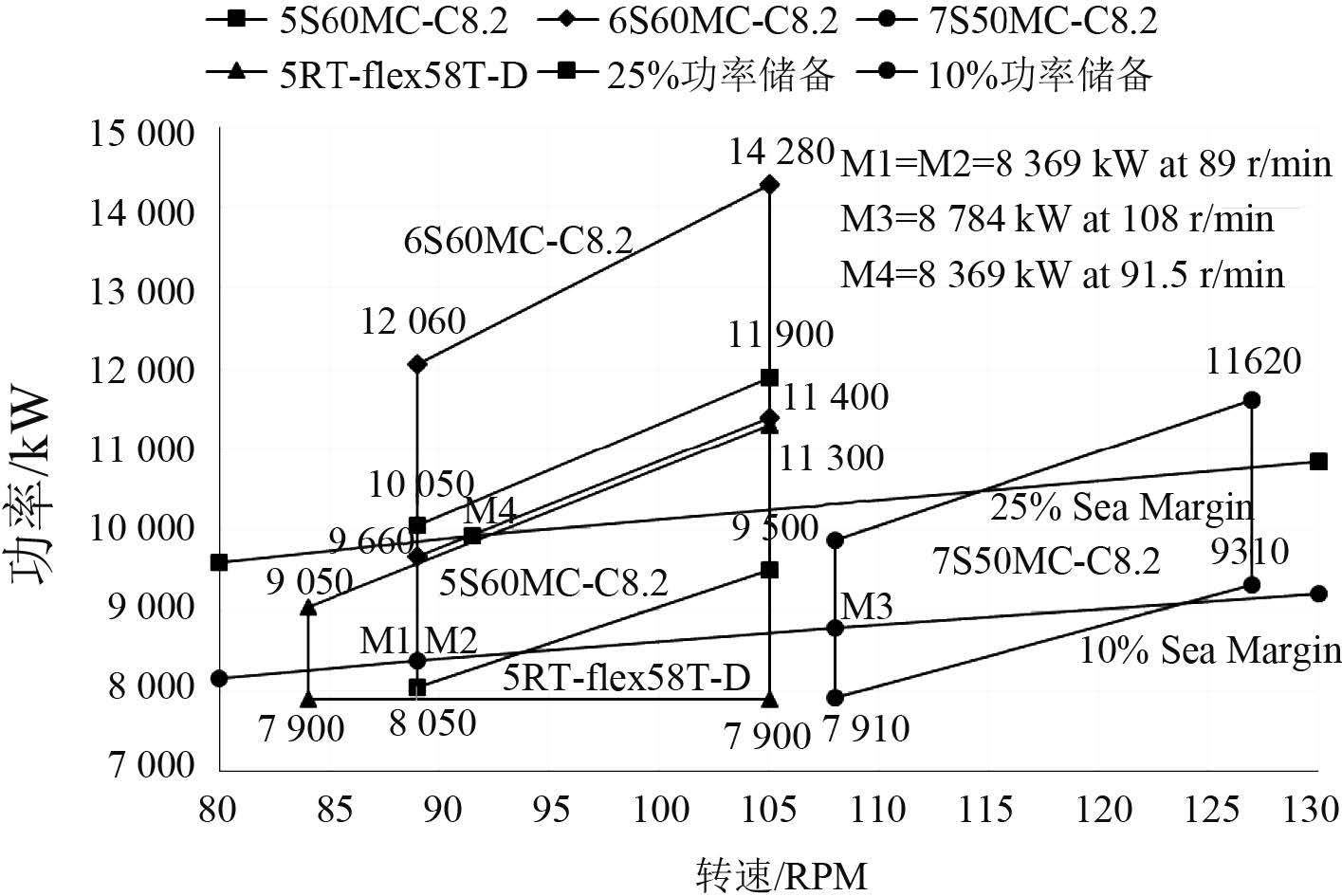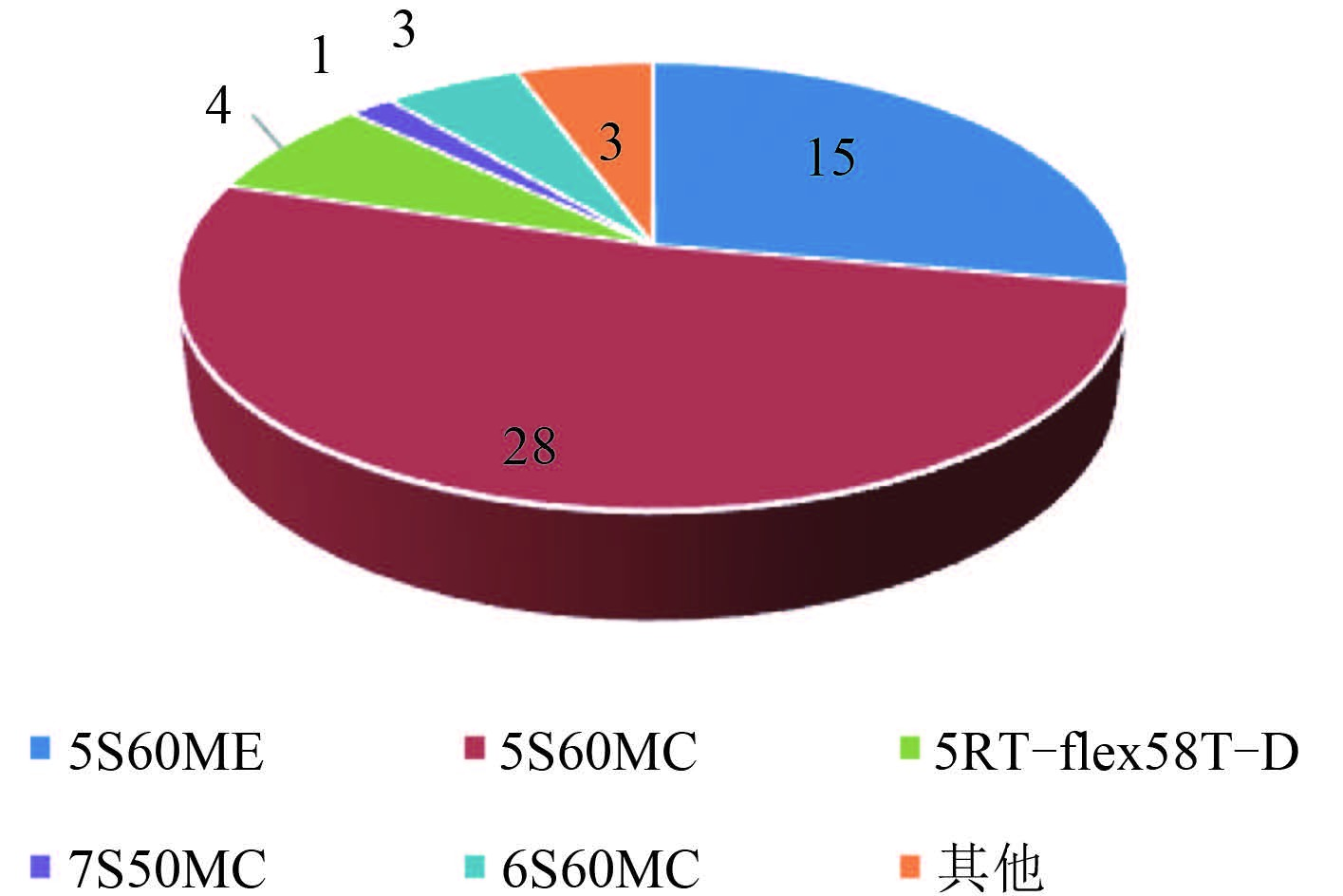﻿ 基于EEDI的船舶主机选型研究
 舰船科学技术2016, Vol. 38Issue (5): 96-100,104PDF

1. 大连海事大学, 辽宁 大连 116026 ;
2. 大连船舶重工集团有限公司, 辽宁 大连 116006

Research on marine engine selection based on ship energy efficiency design index
DA Yong1, ZHANG Yue-wen1, ZHANG Peng1, LI Guo-long2
1. Dalian Maritime University, Dalian 116026, China ;
2. Dalian Shipbuilding Industry Co. Ltd., Dalian 116006, China
Abstract: In the background of EEDI, the paper focusing on the research on the EEDI during the process of main engine's selection based on green ship-Dolphin 82 K designed by SDARI.To achieve a scientific, objective and reasonable assessment on the engine's selection, using the EEDI as one of the indicators measuring the quality of engine selection and establishing the comprehensive evaluation model for marine engine selection.
Key words: EEDI     engine selection     fuzzy comprehensive evaluation

1 船舶能效设计指数（EEDI）

EEDI 是用来衡量新造船舶能效水平高低的指标，利用船舶在特定工况下的 CO2 排放水平来表示。把 EEDI 简单理解为新造船单位航速、单位载重吨的CO2排放量，适用于除少数特种船舶外的大部分商船，根据第 63 次 MEPC 会议颁布的 EEDI 计算方法得知其公式如下

 $EEDI=\frac{{{E}_{ME}}+{{E}_{AE}}+{{E}_{PTI}}+{{E}_{eff}}}{{{f}_{i}}.Capacity.{{V}_{ref}}.{{f}_{w}}},$ (1)
 ${{E}_{ME}}=\left( \prod\limits_{j=1}^{n}{{{f}_{j}}} \right)\left( \sum\limits_{i=1}^{nME}{{{P}_{ME(i)}}\cdot }{{C}_{FME(i)}}\cdot SF{{C}_{ME(i)}} \right),$ (2)
 ${{E}_{AE}}={{P}_{AE}}\cdot {{C}_{FAE}}\cdot SF{{C}_{AE}},$ (3)
 $\begin{array}{*{35}{l}} {{E}_{PTI}}=\left( \prod\limits_{j=1}^{n}{{{f}_{j}}}\cdot \sum\limits_{i=1}^{nPTI}{{{P}_{PTI(i)}}}-\sum\limits_{i=1}^{neff}{{{f}_{eff(i)}}}\times {{P}_{AEeff(i)}} \right)\times \\ {{C}_{FAE}}\cdot SF{{C}_{AE}}, \\ \end{array}$ (4)
 ${{E}_{eff}}=\sum\limits_{i=1}^{neff}{{{f}_{eff(i)}}}\cdot {{P}_{eff(i)}}\cdot {{C}_{FME}}\cdot SF{{C}_{ME}}.$ (5)

2 船舶主机选型对 EEDI 产生的影响 2.1 Dolphin 82 K 散货船主机选型

 $\frac{P}{{{P}_{0}}}={{\left( \frac{n}{{{n}_{0}}} \right)}^{\alpha }}.$ (6)图 1 82000 DWT 散货常用主机型号选型区域 Fig. 1 Selection area of 82000 DWT common engine type表 1 主机选型对 EEDI 的影响 Tab.1 Influence of engine selection on EEDI

2.2 设计航速

EEDI 对船舶设计航速非常敏感，由海军常数公式（7）可知，船舶主机功率与航速的三次方成正比，当船舶设计航速发生变化时将对 EEDI 产生非常大的影响，且设计航速变化直接影响船舶所选择的主机型号。

 $P=\frac{{{\Delta }^{\frac{2}{3}}}\cdot {{V}^{3}}}{{{C}_{e}}}.$ (7)表 2 设计航速对 EEDI 的影响研究 Tab.2 Influence of design speed on EEDI
2.3 船舶载重量

2.4 船舶所需功率

3 基于 EEDI 的船舶主机选型综合评价模型研究 3.1 模糊综合评价方法基本理论

3.2 船舶主机选型综合评价实例 3.2.1 建立评价因素集

1）从功率和转速的角度，应选择大功率、低转速的主机，以得到较高的船舶动力性及推进效率；

2）从主机初始投资，寿命及可靠性的角度，要求主机造价低，使用寿命长，可靠性高，且维修保养方便；

3）从燃油和滑油消耗的角度，应选择对燃油和滑油品质要求低，且耗油率也低的主机，尽可能提高营运经济性；

4）从重量和尺寸的角度，应选择重量轻，尺寸小的主机，以提升船舶载重量，减小机舱容积、方便机舱布置；

5）所选主机振动噪声应尽量小，以提高机舱工作环境；还要考虑柴油机热效率要高，技术先进成熟，运行可靠等。

 $U=\left\{ {{u}_{1}},{{u}_{2}},{{u}_{3}},{{u}_{4}},{{u}_{5}},{{u}_{6}},{{u}_{7}} \right\}.$} (8)表 3 “某专家”指标权重打分调查表 Tab.3 Index weight scale questionnaire of “Some Expert”

 $\begin{array}{*{35}{l}} A=\left\{ {{a}_{1}},{{a}_{2}},\cdots ,{{a}_{7}} \right\}=\left\{ 0.204,0.155,0.216,0.247, \right. \\ \left. 0.102,0.044,0.032. \right\} \\ \end{array}$ (9)
3.2.2 建立评价方案集表 4 82000 DWT 散货船常用主机机型 Tab.4 Common used engine types of 82000 DWT bulk carrier

 $P=\left\{ {{p}_{1}},{{p}_{2}},{{p}_{3}},{{p}_{4}},{{p}_{5}} \right\}.$ (10)

3.2.3 评价指标标准化及构建模糊综合评价矩阵

 ${{r}_{ij}}=\frac{x\left( i,j \right)-{{x}_{\min }}\left( i,j \right)}{{{x}_{\max }}\left( i,j \right)-{{x}_{\min }}\left( i,j \right)},\left( i=1,2,\cdots ,n\text{?}\!\!\gg\!\!\text{ }j=1,2,\cdots ,m \right);$ (11)

 ${{r}_{ij}}=\frac{{{x}_{\max }}\left( i,j \right)-x\left( i,j \right)}{{{x}_{\max }}\left( i,j \right)-{{x}_{\min }}\left( i,j \right)},\left( i=1,2,\cdots ,n\text{?}\!\!\gg\!\!\text{ }j=1,2,\cdots ,m \right).$ (12)表 5 标准化结果 Tab.5 Standardized results

 $R=\left[ \begin{matrix} {{R}_{1}} \\ {{R}_{2}} \\ {{R}_{3}} \\ {{R}_{4}} \\ {{R}_{5}} \\ {{R}_{6}} \\ {{R}_{7}} \\ \end{matrix} \right]=\left[ \begin{matrix} 0.88 &0.56 &0.0 &1.0 &0.97 \\ 0.0 &0.0 &1.0 &0.0 &0.0 \\ 1.0 &0.68 &0.0 &0.58 &0.44 \\ 0.47 &0.0 &0.72 &1.0 &0.86 \\ 0.50 &0.25 &0.0 &1.0 &0.75 \\ 0.0 &1.0 &0.14 &0.16 &0.44 \\ 0.0 &1.0 &0.09 &0.26 &0.21 \\ \end{matrix} \right].$ (13)
3.2.4 主机选型综合评价

 \begin{align} &B=\left[ {{a}_{1}},{{a}_{2}},\cdots ,{{a}_{7}} \right]{}^\circ \left[ \begin{matrix} {{r}_{11}} &{{r}_{12}} &\cdots &{{r}_{15}} \\ {{r}_{21}} &{{r}_{22}} &\cdots &{{r}_{25}} \\ \vdots &\vdots &\vdots &\vdots \\ {{r}_{71}} &{{r}_{72}} &\cdots &{{r}_{75}} \\ \end{matrix} \right]= \\ &\left[ 0.204,0.155,0.216,0.247,0.102,0.044,0.032 \right]\ \\ &\left[ \begin{matrix} 0.88 &0.56 &0.0 &1.0 &0.97 \\ 0.0 &0.0 &1.0 &0.0 &0.0 \\ 1.0 &0.68 &0.0 &0.58 &0.44 \\ 0.47 &0.0 &0.72 &1.0 &0.86 \\ 0.50 &0.25 &0.0 &1.0 &0.75 \\ 0.0 &1.0 &0.14 &0.16 &0.44 \\ 0.0 &1.0 &0.09 &0.26 &0.21 \\ \end{matrix} \right]= \\ &\left[ 0.56,0.36,0.34,0.69,0.61 \right] \\ \end{align} (14)
4 主机选型模糊综合评价结果图 2 2009-2013 Dolphin 82 K 散货船交付机型 Fig. 2 Used engine of dolphin82K bulk carrier between 2009 and 2013

  郝金凤, 强兆新, 石俊令, 等. 船舶设计节能减排技术策略[J]. 舰船科学技术 , 2012, 34 (9) :3–10. HAO Jin-feng, QIANG Zhao-xin, SHI Jun-ling, et al. Research on response measures for ship emission reduction and energy saving[J]. Ship Science and Technology , 2012, 34 (9) :3–10.  魏昕, 徐建豪. 船舶节能减排思考[J]. 中国海事 , 2012 (8) :37–39. WEI Xin, XU Jian-hao. Consideration of energy efficiency and emission reduction for ships[J]. China Maritime Safety , 2012 (8) :37–39.  刘晓华, 路少君. 海事船舶节能减排的探讨[J]. 中国海事 , 2010 (11) :57–58. LIU Xiao-hua, LU Shao-jun. Discussion on energy saving and emission reduction for the maritime control vessels[J]. China Maritime Safety , 2010 (11) :57–58.  . Ship SEEMP (Energy Efficiency Management Plan)[J]. Ship Standardization Engineer , 2012 (3) :56–59.  刘雅玲. 新造船能效设计指数介绍及分析[J]. 中国造船 , 2009, 50 (4) :165–170. LIU Ya-ling. Introduction and study of new ship energy efficiency design index (EEDI)[J]. Shipbuilding of China , 2009, 50 (4) :165–170.  Resolution MEPC. 2012 Guidelines on the method of calculation of the attained energy efficiency design index (EEDI) for new ships:212(63)[S]. London:IMO, 2012.  张运杰. 模糊数学基础[M]. 大连: 大连海事大学数学系, 1996 . ZHANG Yun-jie. Fuzzy mathematics foundation[M]. Dalian: Department of Mathematics, Dalian Maritime University, 1996 .  陈可越. 船舶设计实用手册总体分册[M]. 北京: 中国交通科技出版社, 2007 . CHEN Ke-yue. Ship design manual[M]. Beijing: China Traffic Science and Technology Press, 2007 .  单卓. MAN B &W ME-GI柴油机双燃料系统风险评估研究[D]. 大连:大连海事大学, 2008. SHAN Zhuo. Study on hazard evaluation for dual fuel system of MAN B &W ME-GI engine[D]. Dalian:Dalian Maritime University, 2008. http://cdmd.cnki.com.cn/Article/CDMD-10151-2008210108.htm  陈守煜. 论相对隶属度[J]. 大自然探索 , 1993, 12 (2) :25–27. CHEN Shou-yu. The relative membership degree[J]. Exploration of Nature , 1993, 12 (2) :25–27.